# JP4465570B2 - Image processing apparatus and method, and recording medium - Google Patents

## Info

Publication number
JP4465570B2
JP4465570B2 JP2000292316A JP2000292316A JP4465570B2 JP 4465570 B2 JP4465570 B2 JP 4465570B2 JP 2000292316 A JP2000292316 A JP 2000292316A JP 2000292316 A JP2000292316 A JP 2000292316A JP 4465570 B2 JP4465570 B2 JP 4465570B2
Authority
JP
Japan
Prior art keywords
interpolation
input
video signal
video signals
format
Prior art date
Legal status (The legal status is an assumption and is not a legal conclusion. Google has not performed a legal analysis and makes no representation as to the accuracy of the status listed.)
Active
Application number
JP2000292316A
Other languages
Japanese (ja)
Other versions
JP2002101336A (en
Inventor

Original Assignee
ソニー株式会社
Priority date (The priority date is an assumption and is not a legal conclusion. Google has not performed a legal analysis and makes no representation as to the accuracy of the date listed.)
Filing date
Publication date
Application filed by ソニー株式会社 filed Critical ソニー株式会社
Priority to JP2000292316A priority Critical patent/JP4465570B2/en
Publication of JP2002101336A publication Critical patent/JP2002101336A/en
Application granted granted Critical
Publication of JP4465570B2 publication Critical patent/JP4465570B2/en
Application status is Active legal-status Critical
Anticipated expiration legal-status Critical

## Images

•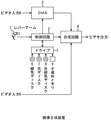•••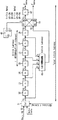•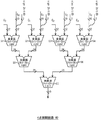•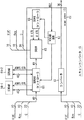•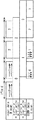•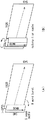•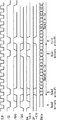••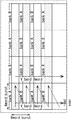•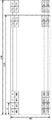•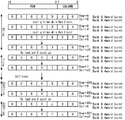•••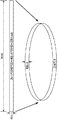•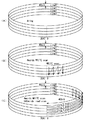•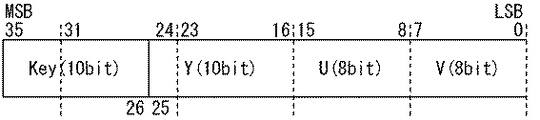•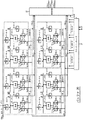•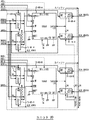•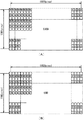•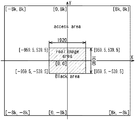••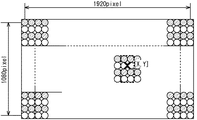•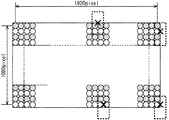•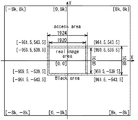•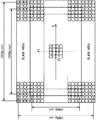•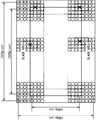•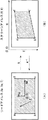•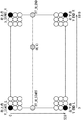•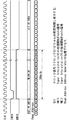•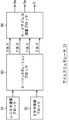••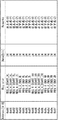•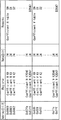••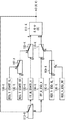•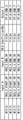•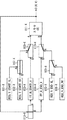•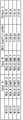•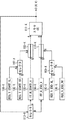•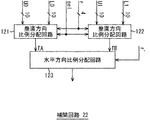•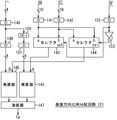••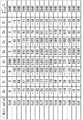•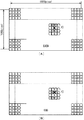•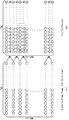•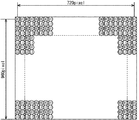•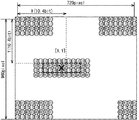•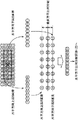•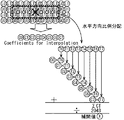••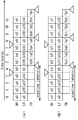•## Classifications

• GPHYSICS
• G06COMPUTING; CALCULATING; COUNTING
• G06TIMAGE DATA PROCESSING OR GENERATION, IN GENERAL
• G06T3/00Geometric image transformation in the plane of the image
• G06T3/40Scaling the whole image or part thereof
• G06T3/4007Interpolation-based scaling, e.g. bilinear interpolation
• HELECTRICITY
• H04ELECTRIC COMMUNICATION TECHNIQUE
• H04NPICTORIAL COMMUNICATION, e.g. TELEVISION
• H04N5/00Details of television systems
• H04N5/222Studio circuitry; Studio devices; Studio equipment ; Cameras comprising an electronic image sensor, e.g. digital cameras, video cameras, TV cameras, video cameras, camcorders, webcams, camera modules for embedding in other devices, e.g. mobile phones, computers or vehicles
• H04N5/262Studio circuits, e.g. for mixing, switching-over, change of character of image, other special effects ; Cameras specially adapted for the electronic generation of special effects
• H04N5/2628Alteration of picture size, shape, position or orientation, e.g. zooming, rotation, rolling, perspective, translation
• HELECTRICITY
• H04ELECTRIC COMMUNICATION TECHNIQUE
• H04NPICTORIAL COMMUNICATION, e.g. TELEVISION
• H04N7/00Television systems
• H04N7/01Conversion of standards, e.g. involving analogue television standards or digital television standards processed at pixel level
• H04N7/0135Conversion of standards, e.g. involving analogue television standards or digital television standards processed at pixel level involving interpolation processes

## Description


BACKGROUND OF THE INVENTION
The present invention relates to an image processing apparatus and method, and a recording medium. For example, the present invention relates to an image processing apparatus and method, and a recording medium that are suitable for use in arbitrarily changing the shape of an image for display.

[Prior art]
The advent of digital storage has greatly contributed to the technical progress of television program production techniques. DRAM (Dinamic Random Access Memory) of digital storage has a recording capacity of one scanning line, one field image, one frame image, and a series of multiple images. It has been gradually increased. Further, even in consideration of the manufacturing cost, circuit scale, power consumption, etc., it is economically practical.

As an application example of digital storage such as DRAM, there is so-called DME (Digital Multi Effects) which is used when an image is deformed or moved to an arbitrary shape when a television program is produced.

By the way, when a conventional DME or the like that processes a video signal in a conventional HD (High Definition) format performs an interpolation process, a video at a position to be interpolated using video signals respectively corresponding to four pixels around the position to be interpolated. A so-called four-point interpolation process for calculating a signal is performed. Also, when a conventional DME or the like that processes an SD (Standered Definition) format video signal performs an interpolation process, after performing field / frame conversion, the position to be interpolated with the horizontal scanning line doubled. A so-called 16-point interpolation process is performed in which video signals at positions to be interpolated are calculated using video signals respectively corresponding to 16 pixels in the vicinity of. Here, if the SD format video signal is subjected to 4-point interpolation, the interpolated video signal is inferior to the case where 16-point interpolation is performed.

[Problems to be solved by the invention]
Therefore, when realizing a DME capable of processing an HD format video signal and an SD format video signal, four-point interpolation can be performed on the HD format video signal, and the SD format video signal is converted into an SD format video signal. On the other hand, it is desirable that 16-point interpolation can be performed, but there is a problem that such DME has not been realized.

The present invention has been made in view of such a situation. Four-point interpolation processing can be executed for an HD format video signal, and for an SD format video signal, 16 points can be executed. An object is to enable point interpolation processing to be executed.

[Means for Solving the Problems]
An image processing apparatus according to the present invention includes a recording unit that records an input video signal in a memory, a reading unit that simultaneously reads a predetermined number of video signals recorded in the memory, and a plurality of reading units read from the memory by the reading unit. Interpolating means that interpolates the video signal corresponding to a predetermined position by performing a predetermined calculation on the video signal, and controlling the operating frequency and the number of operations of the reading means and the interpolating means according to the format of the input video signal And a control means.

When the video signal of the first format is input to the control means, the operating frequency of the reading means and the interpolation means and the number of times of the operation are quadrupled compared to the case where the video signal of the second format is input. Can be changed.

Based on the control from the control means, the interpolation means performs a predetermined calculation on the 16 video signals and interpolates the video signals corresponding to the predetermined positions when the video signals of the first format are input. When video signals of the second format are input, a predetermined calculation can be performed on the four video signals to interpolate the video signals corresponding to the predetermined positions.

The image processing apparatus of the present invention can further include conversion means for performing field frame conversion on the video signal of the first format and doubling the number of pixels in the vertical direction.

The image processing method according to the present invention includes a recording step of recording an input video signal in a memory, a reading step of simultaneously reading out a predetermined number of video signals recorded in the memory, and a reading step of reading from the memory. An interpolation step for performing a predetermined calculation on the plurality of video signals and interpolating a video signal corresponding to a predetermined position, and a read step process and an interpolation step process corresponding to the format of the input video signal And a control step for controlling the operation frequency and the number of operations.

The recording medium program of the present invention includes a recording step for recording an input video signal in a memory, a reading step for simultaneously reading a predetermined number of video signals recorded in the memory, and reading from the memory by the processing of the reading step. An interpolation step for performing a predetermined operation on the output video signals and interpolating a video signal corresponding to a predetermined position, and a read step process and an interpolation step corresponding to the format of the input video signal And a control step for controlling the operation frequency and the number of operations of the process.

In the image processing apparatus and method and the recording medium program of the present invention, an input video signal is recorded in a memory, and the video signals recorded in the memory are simultaneously read out every predetermined number. In addition, a predetermined calculation is performed on the plurality of video signals read from the memory, and the video signal corresponding to the predetermined position is interpolated. Note that the operation frequency and the number of operations of read processing and interpolation processing are controlled in accordance with the format of the input video signal.

DETAILED DESCRIPTION OF THE INVENTION
An image composition apparatus to which the present invention is applied will be described with reference to FIG. FIG. 1 is a block diagram illustrating a configuration example of an image composition apparatus. This image composition device is used, for example, in the production of a television broadcast program, and synthesizes a video input A image and a video input B image that have undergone digital image processing such as deformation and movement. Output.

The image synthesizing apparatus detects a user's operation and outputs a corresponding operation signal to the control circuit 2 and controls the drive 5 to control a magnetic disk 6 (including a floppy disk), an optical disk 7 (CD-ROM ( The control program stored in the compact disc-read only memory (DVD) (including digital versatile disc), magneto-optical disk 8 (including MD (mini disc)), or semiconductor memory 9 is read and read. A control circuit 2 for controlling the entire image synthesizing apparatus based on an output control program, an operation signal from the lever arm 1, etc., a DME 3 for performing digital image processing on the video input A and outputting it to the synthesizing circuit 4; The video input A video is superimposed on the video input A video that has undergone digital image processing and output to the subsequent stage.

Next, the operation of the image composition device will be described. The video of the video input A is subjected to digital image processing corresponding to the user's operation on the lever arm 1 by the DME 3 and is superimposed on the video of the video input B by the synthesis circuit 4 and output.

FIG. 2 shows a configuration example of the DME 3. The video input A input to the DME 3 is a video signal (30 bits wide) in an HD format (eg, 1080i × 1920) of 4: 2: 2: 4 (Y / U / V / K), that is, 10 bits wide Image signal composed of a luminance signal Y, color difference signals U and V each having a width of 5 bits, and a key signal K having a width of 10 bits. As the video input A, an HD (High Definition) format video signal, an SD (Standered Definition) format video signal (for example, 480i × 720), and other format video signals can be input. It is.

In FIG. 2, the luminance signal Y is displayed as a signal Y, the 5-bit wide color difference signals U and V are integrated and displayed as a 10-bit wide C signal, and the key signal K is displayed as a K signal. ing.

A horizontal defocus filter (hereinafter referred to as HDFF (Horizontal Defocus Filter)) 11 of the DME 3 is an H filter 12 that performs one-dimensional low-pass filter processing on the luminance signal Y input in the horizontal scanning order, and in the horizontal scanning order. After interpolating the H filter 13 that performs one-dimensional low-pass filter processing on the input key signal K and the color difference signals U and V input in the order of horizontal scanning (see FIGS. 3 to 6 for details) It is configured by an H filter 14 that performs one-dimensional low-pass filter processing (described later). Therefore, the 4: 4: 4: 4 video signal (40-bit width) is supplied to the scan converter 15 subsequent to the HDFF 11.

The scan converter 15 holds video signals input from the HDFF 11 in the order of horizontal scanning, scans the held video signals in the vertical direction, that is, converts the scanning direction from the horizontal direction to the vertical direction, and The image is output to a focus filter (hereinafter referred to as VDFF (Vertical Defocus Filter)) 16.

Further, the scan converter 15 reduces the bit width of the color difference signals U and V in the video signal (40-bit width) returned from the VDFF 16 in the order of the vertical scanning and outputs the reduced signal to the buffer 20.

Further, when the video signal returned from the VDFF 16 is in the SD format, the scan converter 15 converts the field image into a frame image, interpolates, and outputs the frame image to the buffer 20. When the video signal input from the VDFF 16 is in the HD format, it is output to the buffer 20 in the state of a field image.

The VDFF 16 is a V filter 17 that performs one-dimensional low-pass filter processing on the luminance signal Y input in the vertical scanning order, a V filter 18 that performs one-dimensional low-pass filtering processing on the key signal K input in the vertical scanning order, and The color difference signals U and V inputted in the order of vertical scanning are constituted by a V filter 19 which applies a one-dimensional low-pass filter process. The VDFF 16 returns the 4: 4: 4: 4 video signal (40-bit width) subjected to the one-dimensional low-pass filter processing in the vertical direction to the scan converter 15.

The buffer 20 is composed of ZBT SRAM (Zero Bus Turnaround Static Random Access Memory), writes the video signal supplied from the scan converter 15 in accordance with the write address supplied from the address generator 21, and the address generator 21. Are read out in units of four pixels in accordance with the read address (read adrs) supplied from, and output to the interpolation circuit 22.

The interpolation circuit 22 uses the video signal input from the buffer 20 in units of 4 pixels, interpolates the video signal corresponding to a predetermined position inside the 4 pixels, and outputs it to the synthesis circuit 4.

The address generator 15 and the interpolation circuit 22 may be configured by an FPGA (Field Programmable Gate Array).

Next, the color difference signals U and V of the 4: 2: 2: 4 video signal input to the HDFF 11 are interpolated by the H filter 14 and converted to a 4: 4: 4: 4 video signal. This will be described with reference to FIGS. In the following description, the notation regarding the key signal K is omitted, and it is also described as a 4: 2: 2 (Y / U / V) video signal or a 4: 4: 4 video signal.

FIG. 3 shows a concept of processing for interpolating the color difference signals U and V.

FIG. 3A shows a video signal input to the HDFF 11 in the order of horizontal scanning. That is, the HDFF 11 has a luminance signal Y corresponding to a certain pixel (the 0th pixel).0And the color difference signal U corresponding to the 0th pixel0Are input simultaneously. In the next clock, the luminance signal Y corresponding to the 0.5th pixel located to the right of 0th0.5And the color difference signal V corresponding to the 0th pixel.0Are input simultaneously. In the next clock, the luminance signal Y corresponding to the first pixel located to the right of the 0.5th1And the color difference signal U corresponding to the first pixel1Are input simultaneously. Similarly, the luminance signal Y corresponding to the Nth pixelNAnd the color difference signal U corresponding to the Nth pixelNAre input at the same time, and in the next clock, the luminance signal Y corresponding to the (N + 0.5) th pixel located on the right side of the NthN + 0.5And the color difference signal V corresponding to the Nth pixel.NAre input simultaneously.

As shown in FIG. 3A, the luminance signal Y corresponding to the Nth pixel.NAnd color difference signal UN, VNAre not simultaneously input, and the color difference signal U corresponding to the (N + 0.5) th pixelN + 0.5, VN + 0.5Does not exist. Therefore, the luminance signal YNAnd color difference signal UN, VNAnd the color difference signal U corresponding to the (N + 0.5) th pixel in order to unify the spatial frequency of the luminance signal and the color difference signal.N + 0.5, VN + 0.5Is interpolated.

Color difference signal U corresponding to the (N + 0.5) th pixelN + 0.53B, as shown in FIG. 3B, the color difference signals of the pixels adjacent to the left and right, that is, the color difference signal U corresponding to the (N-1) th pixel.N-1, The color difference signal U corresponding to the Nth pixelN, The color difference signal U corresponding to the (N + 1) th pixelN + 1, And the color difference signal U corresponding to the (N + 2) th pixelN + 2Interpolate using.

Similarly, the color difference signal V corresponding to the (N + 0.5) th pixelN + 0.5As shown in FIG. 3C, the color difference signals of the pixels adjacent to the left and right, that is, the color difference signal V corresponding to the (N−1) th pixel are interpolated.N-1, The color difference signal V corresponding to the Nth pixelN, The color difference signal V corresponding to the (N + 1) th pixelN + 1, And the color difference signal V corresponding to the (N + 2) th pixelN + 2Interpolate using.

Next, FIG. 4 shows a configuration example of a portion related to the process of interpolating the color difference signals U and V of the H filter 14.

The selector 31 outputs the color difference signals U and V sequentially input from all stages to the delay circuit (D) 32 and the four-point interpolation circuit 40 in synchronization with the clock. The delay circuits 32 to 37 and 42 delay the color difference signal input from the previous stage by one clock cycle and output it. The delay circuits 33, 35, and 37 also output the color difference signal delayed by one clock cycle to the four-point interpolation circuit 40.

When the control signal S from the D flip-flop 41 is 0, the selector 39 outputs the output of the delay circuit 38 input to its own DA terminal from the QA terminal to the delay circuit 42 and also to its own DB terminal. The output of the input 4-point interpolation circuit 40 is output from the QB terminal. On the other hand, when the control signal S from the D flip-flop 41 is 1, the output of the delay circuit 38 input to its own DA terminal is output from the QB terminal and is input to its own DB terminal. The output of the interpolation circuit 40 is output from the QA terminal to the delay circuit 42. The selector 39 receives the color difference signal U as shown in FIG.NAnd color difference signal VN-0.5Are output at the same time.

The 4-point interpolation circuit 40 interpolates the color difference signal by pipeline processing that requires 4 clock cycles using the following equation.
Interpolated color difference signal value
= (T0* C0+ T1* C1+ T2* C2+ TThree* CThree) / (C0+ C1+ C2+ CThree)

Where t0Is the color difference signal U corresponding to the (N−1) th pixel inputted from the delay circuit 37.N-1(Or VN-1) And t1Is the color difference signal U corresponding to the Nth pixel input from the delay circuit 35.N(Or VN) And t2Is the color difference signal U corresponding to the (N + 1) th pixel input from the delay circuit 33.N + 1(Or VN + 1) And tThreeIs the color difference signal U corresponding to the (N + 2) th pixel input from the selector 31.N + 2(Or VN + 2) Value. Here, the interpolation coefficient C0Thru CFourAre −163, 1187, 1187, and −163, respectively.

The D flip-flop 41 alternately outputs 0 and 1 as the control signal S to the selector 39 every clock.

FIG. 5 shows a configuration example of the four-point interpolation circuit 40. The four-point interpolation circuit 40 includes multipliers 51 to 54 and adders 55 to 57.

The multiplier 51 receives the color difference signal U corresponding to the (N−1) th pixel input from the selector 31.N-1(Or VN-1) Value t0Interpolation coefficient C0Multiplication value t multiplied by0* C0Is output to the adder 55. The multiplier 52 receives the color difference signal U corresponding to the Nth pixel input from the delay circuit 33.N(Or VN) Value t1Interpolation coefficient C1Multiplication value t multiplied by1* C1Is output to the adder 55. The multiplier 53 receives the color difference signal U corresponding to the (N + 1) th pixel input from the delay circuit 35.N + 1(Or VN + 1) Value t2Interpolation coefficient C2Multiplication value t multiplied by2* C2Is output to the calculator 56. The multiplier 54 receives the color difference signal U corresponding to the (N + 2) th pixel input from the delay circuit 37.N + 2(Or VN + 2) Value tThreeInterpolation coefficient CThreeMultiplication value t multiplied byThree* CThreeIs output to the calculator 56.


Next, the operation of the functional block related to the process of interpolating the color difference signals U and V will be described.

For example, as shown in FIG. 4, at the clock timing t0, the selector 31, the delay circuit 32 to the delay circuit 38 are respectively connected to the color difference signal V.Four, UFour, VThree, UThree, V2, U2, V1, U1Is output to the subsequent stage, the color difference signal V is obtained by the four-point interpolation circuit 40 in the subsequent four clock cycles.2.5Is interpolated. Therefore, at the clock timing t4, the color difference signal U is sent from the delay circuit 38 to the DA terminal of the selector 39.ThreeThe color difference signal V interpolated by the 4-point interpolation circuit 40 is input to the DB terminal.2.5Is entered.

At this time, the selector 39 corresponds to the control signal S = 0 from the D flip-flop 41, and the color difference signal U input to the DA terminal.ThreeFrom the QA terminal to the delay circuit 42 and the interpolated color difference signal V input to the DB terminal.2.5Is output from the QB terminal. In synchronism with this, from the delay circuit 42, the color difference signal U which has been interpolated and delayed by one clock before.2.5Is output. Therefore, from the H filter 14, as shown in FIG. 3E, the color difference signal U corresponding to the (N + 0.5) th pixel.N + 0.5And color difference signal VN + 0.5Will be output at the same time.

As described above, the HDFF 11 removes the high-frequency component of the 4: 2: 2 video signal and interpolates the color difference signal to convert it to a 4: 4: 4 video signal. The luminance signal Y and the color difference signals U and V can be handled at the same spatial frequency. Thereby, for example, as a process related to the color operation of the video, it is possible to perform a colored spotlight process, a trail process with a color change, and the like.

Next, FIG. 6 shows a configuration example of the scan converter 15 that converts the scanning direction of the 4: 4: 4 video signal input from the HDFF 11 in the order of horizontal scanning from the horizontal direction to the vertical direction. The scan converter 15 includes a scan conversion IC 61 made of an FPGA or the like, and SRAMs (Synchronous Dynamic Random Access Memory) 64-1 and 64-2.

The V scan generator 62 of the scan conversion IC 61 generates a signal indicating the corresponding vertical scanning timing based on the horizontal scanning timing indicated by the REF signal supplied from the outside, and outputs the signal to the SDRAM controller 63 and the SRAM controller 66.

The SDRAM controller 63 switches the 4: 4: 4: 4 video signal input from the HDFF 11 in units of fields and records them in the SDRAMs 64-1 and 64-2. The SDRAM controller 63 also reads the video signals recorded in the SDRAMs 64-1 and 64-2 in a predetermined order (described later) and outputs them to an SRAM (Static Random Access Memory) 65.

The SRAM 65 has a capacity of 2 bits * 2048 per block, and uses four memories of 20 blocks. Based on the control of the SRAM controller 66, the SRAM 65 caches a video signal input from the SDRAM controller 63. Output to VDFF16.

The converter 67 reduces the information amount of the color difference signals U and V in the video signal input from the VDFF 16 in the vertical scanning order from 10-bit width to 8-bit width (see FIG. 18 for details). Will be described later). When the video signal input from the VDFF 16 is in the SD format, the converter 67 converts the field image into a frame image (details will be described later with reference to FIG. 47).

FIG. 7 shows a rough time transition of the process of converting the scanning direction to vertical in field units. In the following figures, buffer A corresponds to one of SDRAMs 64-1 and 64-2, and buffer B corresponds to the other.

FIG. 8 schematically shows the relationship between the SDRAMs 64-1 and 64-2 that burst-transfer video signals recorded in field units in the vertical scanning order, and the SRAM 65 that caches the burst-transferred video signals. ing. That is, the SRAM 65 operates as if moving horizontally from the left to the right of the image like a caterpillar while scanning the video signals recorded in the SDRAMs 64-1 and 64-2 in the vertical direction. Cache the signal. If the transfer amount per unit time input to the SDRAMs 64-1 and 64-2 in the horizontal scanning order is equal to the transfer amount per unit time output to the SRAM 65 in the vertical scanning order, the system is stable. Operation is guaranteed. That is, in the SDRAMs 64-1 and 64-2, a situation where the read address overtakes the write address does not occur.

Note that the SDRAMs 64-1 and 64-2 (hereinafter simply referred to as the SDRAM 64 when there is no need to distinguish between the SDRAMs 64-1 and 64-2) have a plurality of (for example, two types) SDRAM characteristics. When burst transfer with an appropriate width (auto pre-charge 4 word burst) is alternately performed on a bank, continuous access (read or write) is guaranteed. FIG. 9 shows an example of the timing of successive access (write burst) in alternating bursts for two types of banks using such characteristics.

Specifically, as shown in FIG. 10, the video signal written in the horizontal scanning order to the SDRAM 64 is burst-transferred in units of 8 words, that is, 4 words to each bank. As shown in FIG. 11, the video signal read from the SDRAM 64 in the order of vertical scanning is also burst-transferred in units of 8 words, that is, 4 words from each bank.

Here, one word indicates a 40-bit information amount indicating a luminance signal Y (10 bits), color difference signals U and V (10 bits each), and a key signal K (10 bits) corresponding to one pixel. ing.

As described above, the video signal cached by burst transfer to the SRAM 65 in units of 8 words (for two rectangular areas in FIG. 11) is read from the SRAM 65 after being delayed by a time corresponding to four vertical scans. For example, the SRAM 65 can have a minimum capacity (capacity corresponding to 0.4% of one field for two rectangular areas in FIG. 11).

FIG. 12 shows an example of two-dimensional allocation of addresses in a 2-bank 4-word burst of an HD format (1080i × 1920) video signal to the SDRAM 64.

As shown in the figure, at the time of writing, the burst head address is controlled so that writing is performed at an address in accordance with horizontal scanning. In this case, at the time of reading, it is accessed as a band having a burst size width.

A write address for the SDRAM 64 is generated by a counter mechanism including an upper counter (ROW) and a lower counter (COLUMN) as shown in FIG.

(1) Count-up is performed by a state machine that outputs a 4-word burst once every two times. The next count is performed during the bank access on the late side.
(2) Counter reload and 2K up are performed at 0x3c0. For each lower 0x3c0 count, 1k is added to the reload data.
(3) When the value of the upper counter reaches 540, one field is completed.

FIG. 14 shows the order of continuous reading from the SDRAM 64. As shown in the figure, by using 4-word burst 2bank pingpong access, the writing area to the SRAM 65 is a strip unit (rectangular area (4 words) consisting of 540 rectangular areas in FIG. 11). (n): n = 1, 2,..., 1df) and must be performed continuously.

The generation of the read address from the SDRAM 64 is performed by a counter mechanism including an upper counter (ROW) and a lower counter (COLUMN) as shown in FIG.

(1) The lower counter is reloaded every time. The reload value is incremented by 4 words when the value of the upper counter reaches 540.
(2) When the value of the lower counter reaches 0x3c0 and the value of the upper counter reaches 540, the process is terminated.
(3) Reloading of the upper counter and 2K up are performed every 4 word burst ping-pong.
(4) When the upper count reaches 540, the reloading of one strip unit (4 words * 540 lines) is completed.

Video signals read from the SDRAM 64 in strip units are accessed after being cached in the SRAM 65. However, since the order of access is constant and complete synchronization, it is not an associative structure like a general-purpose cache, but complete synchronization predictive control.

FIG. 16 shows a concept of using four memories (2 bits * 2048 * 20 blocks) constituting the SRAM 65 as a ring having a width of 40 bits (1 word) * 2048. In practice, as shown in FIG. 17A, four rings shown in FIG. 16 are stacked to form a caterpillar (FIG. 8B) having a width of 160 bits (4 words) * 2048.

Specifically, the rectangular areas (4 words) read from the SDRAM 64 in the order shown in FIG. 15 are written sequentially into the rings 0 to 3 one by one as shown in FIG. 17B, for only three vertical scans. Delay and read out in the circumferential direction of the ring as shown in FIG.

Through the operation as described above, the scanning direction of the video signal can be converted from the horizontal direction to the vertical direction in real time, and can be output to the VDFF 16 at the subsequent stage.

As described above, the video signal input to the VDFF 16 is subjected to vertical one-dimensional low-pass filter processing, input to the scan converter 15 again, and supplied to the converter 67.

Next, for the purpose of adapting to the buffer 20 in which eight 36-bit ZBT SRAMs in the subsequent stage are used, the 40-bit width of 4: 4: 4: 4 inputted from the VDFF 16 in the order of vertical scanning is used. Processing of the converter 67 that converts the video signal into a 36-bit video signal will be described.

As shown in FIG. 18, the converter 67 is a video signal (10-bit luminance signal Y, 10-bit color difference signal U, 10-bit luminance signal Y, 4: 4: 4: 4) that is input from the VDFF 16 in the order of vertical scanning. Among the 10-bit color difference signal V and the 10-bit key signal K), the values of the color difference signals U and V that finally return the spatial frequency characteristics to ½ are obtained by, for example, rounding down or rounding off. The video signal (Y / U / V / K) is converted to a 36-bit width by reducing each to 8 bits and output to the buffer 20 at the subsequent stage.

Note that the reduction of the bit widths of the color difference signals U and V is not limited to the 8-bit width as described above. For example, the color difference signal U is reduced to 9 bits and the color difference signal is reduced. The reduction range may be changed as appropriate, for example, V is reduced to 7 bits.

As described above, in the converter 67, the video signal (without reducing the bit width) without deteriorating the information amount of the luminance signal Y of the video signal and the key signal K important in the digital video effect. The bit width of (Y / U / V / K) can be adapted to the subsequent buffer 20 (36-bit width ZBT SRAM).

Next, FIG. 19 shows a detailed configuration example of the buffer 20. The buffer 20 includes four units U0, U1, L0, and L1 that can be read simultaneously. A configuration example of the unit U0 is shown in FIG. The unit U0 is configured as a double buffer with an A buffer composed of SRAM 73-U0-A and a B buffer composed of SRAM 73-U0-B. Thereby, the unit U0 realizes two-dimensional reading and simultaneous writing. Similarly, each of the units U0 to L1 has a double buffer configuration, and two-dimensional reading and simultaneous writing are realized. Note that SRAM 73-U0-A to 73-L1-B are simply referred to as SRAM 73 when it is not necessary to distinguish them individually.

FIG. 21 shows allocation of video signals input from the scan converter 15 to the units U0 to L1. That is, when the video signal of the EVEN field output from the scan converter 15 is written into the buffer 20, as shown in FIG. 4A, the mth (m = 0, 2, 4,...) Horizontal Four pixels composed of two adjacent pixels on the scanning line and two adjacent pixels on the (m + 2) th horizontal scanning line immediately below are written in the A buffers of different units U0 to L1, respectively. When the video signal of the ODD field output from the scan converter 15 is written to the buffer 20, as shown in FIG. 5B, the (m + 1) th (m = 0, 2, 4,...) Horizontal Four pixels composed of two adjacent pixels on the scanning line and two adjacent pixels on the m + 3th horizontal scanning line immediately below are written in the B buffers of different units U0 to L1.

In this way, by writing the four pixels adjacent vertically and horizontally to different units U0 to L1, they can be read out simultaneously, so that the pixel located at the center of the four pixels is interpolated using the four-pixel video signal. The processing to be performed can be executed efficiently.

Next, in the effective access area (access area) of the buffer 20, the out-of-area data band (black area) set around the data area (real image area) in which the video signal is written will be described with reference to FIGS. I will explain.

FIG. 22 shows a coordinate system of read addresses (also described as linear addresses) set in the buffer 20, and FIG. 23 shows the video signal of the EVEN field written in the data area (real image area) of FIG. It shows the state.

In general, when a video signal written in the buffer 20 is read, based on an address on the display (hereinafter referred to as a screen address) when the video signal to which the digital effect is applied in the DME 3 is displayed on the display, The read address of the buffer 20 is determined. However, details of the relationship between the screen address and the read address will be described later with reference to FIG.

When the read address [X, Y] is determined at the position indicated by “x” in FIG. 24, the video signals of the four pixels at the top, bottom, left, and right of the position of the read address [X, Y] are read out and the interpolation circuit. 22, the video signal of the pixel corresponding to the read address [X, Y] is interpolated (however, the interpolation process using four pixels is for the HD format video signal, and the SD format video signal Is applied with an interpolation process using 16 pixels).

By the way, when the position indicated by the mark “x” in FIG. 25 is the read address [X, Y], there are no four pixels above, below, left, and right. Requires different processing. Therefore, when a read address [X, Y] is given, it is necessary to determine whether the read address [X, Y] can be applied with normal interpolation processing. Necessary. Therefore, an out-of-area data band is set in the image buffer 20 for the purpose of omitting such a determination circuit.

Specifically, as shown in FIGS. 26 and 27, in the effective access area (access area) of the buffer 20, dummy for two pixels is provided on each of the upper, lower, left and right sides of the data area (real image area) into which the video signal is written. The out-of-area data band (black area) is set by writing the video signal. FIG. 27 shows a state in which the video signal of the EVEN field is written in the data area (real image area) of FIG. 26 and an out-of-area data band is set around the video signal.

Here, it is shown that it is possible to set a data area and an out-of-area data band for writing video signals in the buffer 20 in terms of storage capacity.

The buffer 20 is provided with eight SRAMs 73-U0-A to 73-L1-B as shown in FIG. 19, and four of them store the video signal of the field image. However, one effective access area of the SRAM 73 is 256k words = 256 * 1024 words = 262144 words. Since the data area and the out-of-area data band to be written therein are ¼ of the field image video signal (540 × 1920) and the dummy video signal for two pixels in the upper, lower, left and right directions, the necessary capacity is 544 *. 1924/4 = 261664 words, which are completely stored in one effective access area of the SRAM 73. Therefore, an out-of-area data band can be set in the buffer 20 in terms of storage capacity.

In this manner, by setting the data area and the out-of-area data band in the effective access area of the buffer 20, for example, when the position indicated by “x” in FIG. 28 is the read address [X, Y]. However, since there are four pixels on the top, bottom, left, and right, it is possible to apply a normal interpolation process using four pixels. Therefore, when the read address [X, Y] is given, it is not necessary to determine whether or not normal interpolation processing can be applied to the read address [X, Y]. It is possible to omit a dedicated circuit.

The read address [X, Y] that can be generated at this time is
-960.5 <X <960.5
-540.5 <Y <540.5
It is.


Since the screen address (H, V) is obtained by converting the read address (Xm, Ym, T) using the 3 × 3 conversion matrix A, the screen address (H, V) is sequentially scanned. Inverse matrix A of transformation matrix A-1Can be read to calculate the read address (Xm, Ym, T).

Specifically, the read address (Xm, Ym, T) is calculated as shown in the following equation.
[Expression 1]
Effect parameter a11Thru a33Is the inverse matrix A as shown in the following equation:-1Elements.
[Expression 2]
The rotation coefficients p and q of the lighting modulation axis T are p = cos θ and q = sin θ.

Thus, the read address (Xm, Ym, T) is a function value X (H, V), Y (H, V), T (H, V), Z with the screen address (H, V) as a parameter. Calculated using (H, V).

By the way, the read address is calculated for each pixel (clock) of the screen address to be sequentially scanned. For all the pixels of the screen address, the function values X (H, V), Y (H, V), If T (H, V) and Z (H, V) are calculated and the read address is calculated, the amount of calculation becomes enormous, and a circuit dedicated to the calculation is required.

Therefore, as shown in FIG. 30, about the four end points of the screen address, that is, the upper left point (0,0), the lower left point (0,539), the upper right point (1919,0), and the lower right point (1919,539), Function values X (0,0), Y (0,0), T (0,0), Z (0,0), X (0,539), Y (0,539), T (0,539), Z (0,539) , X (1919,0), Y (1919,0), T (1919,0), Z (1919,0), X (1919,539), Y (1919,539), T (1919,539), Z (1919,539) (hereinafter referred to as function values X (0,0) to Z (1919,539)) is calculated, and function values X (H, V) for other pixels of the screen address , Y (H, V), T (H, V), Z (H, V) are interpolated using the function values X (0,0) to Z (1919,539) calculated for the four end points, The corresponding read address is calculated.

Thus, using function values X (0,0) to Z (1919,539) corresponding to the four end points of the screen address, function values X (H, V) and Y (H, V) for other pixels are used. , T (H, V), Z (H, V) is referred to as Super Interpolation, and the upper left point (0,0) and lower left point (0,539) Interpolation processing of the direction, or vertical interpolation processing of the upper right point (1919,0) and lower right point (1919,539) is called super interpolation (V), and the result of super interpolation (V), etc. The horizontal interpolation process using the function values of the left and right end points on the horizontal scanning line is referred to as super interpolation (H).

Next, processing timing of super interpolation will be described with reference to FIG. When super-interpolation is performed for a field image, function values X (0,0) to Z (1919,539) are calculated in advance for each of the four end points of the screen address one field before the field image. It is held in a predetermined register (described later). Then, super-interpolation (V) is executed at the initial stage of the horizontal blanking period (BLANK (H)) in synchronization with the enable of the timing signal VMIX, and the screen address is horizontally scanned in synchronization with the enable of the timing signal HMIX. In the period (ACTIVE AREA), super-interpolation (H) is executed for each clock.

As described above, the super interpolation (H) and the super interpolation (V) have different execution timings.

FIG. 32 shows a configuration example of the address generator 21. The register calculation block 91 calculates the function values X (0,0) to Z (1919,539) at the four end points of the screen address and supplies them to the super interpolation block 93. The mixer coefficient block 92 supplies the mixer coefficient previously stored in the built-in register to the super interpolation block 93.

The super interpolation block 93 receives the function values (0, 0) to Z (1919, 539) of the four end points of the screen address supplied from the register calculation block 91 and the mixer coefficient supplied from the mixer coefficient block 92. Are used to execute super interpolation (H) and super interpolation (V), and function values X (H, V) and Y (H, respectively) corresponding to pixels other than the four end points on the obtained screen address. V), T (H, V), and Z (H, V) are interpolated and output to the read address calculation block 94.

The read address calculation block 94 has function values X (H, V), Y (H, V), T (H, V), T (H, V), respectively corresponding to all pixels of the screen address input from the super interpolation block 93. Using Z (H, V), a read address is generated and output to the buffer 20.

FIG. 33 shows a configuration example of the super interpolation block 93. The super interpolation block 93 is a block that interpolates a function value X (H, V), a block that interpolates a function value Y (H, V), a block that interpolates a function value T (H, V), and a function value Consists of blocks that interpolate Z (H, V).

The REG_V_START_XL register 101-X of the block that interpolates the function value X (H, V) holds the function value X (0,0) for the upper left point (0,0) supplied from the register operation block 91, and the selector Output to the A terminal of 107-X. The REG_V_START_XR register 102 -X holds the function value X (1919,0) for the upper right point (1919,0) supplied from the register operation block 91 and outputs it to the B terminal of the selector 107 -X. The FF_H_START_X register 103-X holds the output of the mixer 111-X input via the A terminal of the selector 112-X and outputs it to the B terminal of the selector 108-X. The FF_H_END_X register 104-X holds the output of the mixer 111-X input via the B terminal of the selector 112-X and outputs it to the B terminal of the selector 110-X. The REG_V_END_XL register 105-X holds the function value X (0,539) for the lower left point (0,539) supplied from the register operation block 91 and outputs it to the B terminal of the selector 109-X. The REG_V_END_XR register 106-X holds the function value X (1919,539) for the lower right point (1919,539) supplied from the register operation block 91 and outputs it to the A terminal of the selector 109-X.

The selectors 107-X to 110-X output the input to the A terminal or the B terminal to the subsequent stage. The selector 112-X outputs the output of the mixer 111-X to the FF_H_START_X register 103-X or the FF_H_END_X register 104-X. The mixer 111 -X sets the output from the selector 108 -X input to the A terminal to A, sets the output from the selector 110 -X input to the B terminal to B, and sets the mixer coefficient supplied from the mixer coefficient block 92 to k.nIn this case, the interpolation value C is output to the subsequent stage using the following equation for each clock.
Interpolated value C = A · (1.0−kn) + B ・ kn
However, the following equation is actually used to decrease the number of multiplications by one.
Interpolated value C = kn(B-A) + A

The configuration of each block that interpolates each of the function values Y (H, V), T (H, V), and Z (H, V) is the same as the configuration of the block that calculates the function value X (H, V). Since it is the same, the description is omitted. However, the correspondence between the REG_V_START_XL registers 101-X to REG_V_END_ZR registers 106-Z and the function values X (0,0) to Z (1919,539) held by them is as shown in FIG.

FIG. 35 shows the correspondence between the registers built in the mixer coefficient block 92 and the mixer coefficients held therein.

Next, the operation of the super interpolation block 93 will be described. It is assumed that corresponding function values (0, 0) to Z (1919, 539) are supplied from the register operation block 91 to the REG_V_START_XL registers 101-X to REG_V_END_ZR registers 106-Z.

First, the vertical component V of the screen address is initialized to V = 0, and super-interpolation (V) is started in synchronization with Enable of the timing signal VMIX. First, in order to execute super interpolation (V) at the left end point (0, V) of the screen address, input sources and output destinations of function values to the mixers 111-X to 111-Z are shown in FIG. Switching is performed in each block so as to be as described above.

Specifically, for example, in a block for interpolating the function value X (H, V), the selectors 107-X to 110-X, 112-X are switched as shown in FIG. As a result, the function value X (0,0) for the upper left point (0,0) held in the REG_V_START_XL register 101-X is input to the A terminal of the mixer 111-X, and the REG_V_END_XL is input to the B terminal. The function value X (0,539) for the lower left point (0,539) held in the register 105-X is input. The mixer coefficient is further supplied from the mixer coefficient supply block 92 to the mixer 111 -X. The mixer 111-X interpolates the function value X (0, V) for the left end point (0, V) of the screen address. The interpolated function value X (0, V) is latched in the FF_H_START_X register 103-X via the selector 112-X.

The same processing is performed in the other blocks, and the function value Y (0, V), function value T (0, V), function value Z (0, V) for the left end point (0, V) of the screen address. ) Are latched in the corresponding FF_H_START_X registers 103-Y to 103-Z, respectively.

Next, in order to execute the super interpolation (V) of the right end point (1919, V) of the screen address, the input source and output destination of the function values to the mixers 111-X to 111-Z are shown in FIG. As shown, switching and the like are performed in each block.

Specifically, for example, in the block for interpolating the function value X (H, V), the selectors 107-X to 110-X, 112-X are switched as shown in FIG. Accordingly, the function value X (1919,539) for the upper right point (1919,539) held in the REG_V_START_XR register 102-X is input to the A terminal of the mixer 111-X, and the REG_V_END_XR is input to the B terminal. The function value X (1919,539) for the lower right point (1919,539) held in the register 106-X is input. The mixer coefficient is further supplied from the mixer coefficient supply block 92 to the mixer 111 -X. The mixer 111-X interpolates the function value X (1919, V) for the right end point (1919, V) of the screen address. The interpolated function value X (1919, V) is latched by FF_H_END_X 104-X via the selector 112-X.

The same processing is performed in the other blocks, and the function value Y (1919, V), function value T (1919, V), function value Z (1919, V) for the right end point (1919, V) of the screen address is performed. ) Are latched in the corresponding FF_H_END_X registers 104-Y to 104-Z, respectively.

The processing so far is executed in the horizontal blanking period.

Thereafter, in synchronization with the Enable of the timing signal HMIX, the horizontal component H of the screen address is initialized to H = 0, and superinterpolation (H) is started. In order to execute super interpolation (H), switching and the like are performed in each block so that the input source and output destination of the function values to the mixers 111-X to 111-Z are as shown in FIG. The

Specifically, for example, in the block that interpolates the function value X (H, V), the selectors 108-X and 110-X are switched as shown in FIG. As a result, the function value X (0, V) for the left end point (0, V) held in the FF_H_START_X register 103-X is input to the A terminal of the mixer 111-X, and the FF_H_END_X is input to the B terminal. The function value X (1919, V) for the right end point (1919, V) held in the register 104-X is input. The mixer coefficient is further supplied to the mixer 111-X from the mixer coefficient supply block 92 for each clock. The mixer 111 -X sequentially interpolates the function value X (H, V) for the right end point (1919, V) from the left end point (0, V) and supplies it to the read address calculation block 94 every clock.

The same processing is performed in other blocks, and the function value Y (H, V) and the function value T (from the left end point (0, V) to the right end point (1919, V) in order for each clock. H, V) and function value Z (H, V) are interpolated and supplied to the read address calculation block 94.

After the horizontal component H of the screen address is initialized, the processing so far is executed in the horizontal scanning period.

Thereafter, the vertical component V is incremented by 1, and the processing after the super interpolation (V) described above is repeated. When the vertical component V reaches 540, super-interpolation with respect to the field being processed is terminated, and the next field is set as a processing target.

As described above, since the super interpolation (V) is executed in the horizontal blanking period and the super interpolation (H) is executed in the horizontal scanning period, the super interpolation (V) and the super interpolation (V) are executed. It becomes possible to execute the interpolation (H) by sharing the same circuit (super interpolation block 93).

Next, the interpolation circuit 22 will be described with reference to FIG. When the video signal buffered in the buffer 20 is in the HD format, the interpolation circuit 22 executes a 4-point interpolation process using a 4-pixel video signal at an operating frequency of 74.25 MHz. Further, when an SD format video signal is field-frame converted and buffered in the buffer 20, a 16-point interpolation process using a 16-pixel video signal is performed at an operating frequency of 54 MHz (an SD format video signal is processed). At a normal operating frequency of 13.5 MHz).

FIG. 42 shows a configuration example of the interpolation circuit 22. The interpolation circuit 22 proportionally distributes the video signals of two pixels adjacent in the vertical direction that are simultaneously input from the units U0 and L0 of the buffer 20, and obtains the interpolation value TA of the video signal corresponding to the position between the two pixels. The vertical proportional distribution circuit 121 to be calculated and the video signals of two pixels adjacent in the vertical direction simultaneously input from the units U1 and L1 of the buffer 20 are proportionally distributed, and the video signal corresponding to the position between the two pixels is distributed. A vertical proportional distribution circuit 122 that calculates the interpolation value TB, and a horizontal proportional distribution that proportionally distributes the interpolation value TA input from the vertical proportional distribution circuit 121 and the interpolation value TB input from the vertical proportional distribution circuit 122. The circuit 123 is configured.

FIG. 43 shows a configuration example of the vertical proportional distribution circuit 121. In the vertical proportional distribution circuit 121, in addition to the video signals of two pixels adjacent in the vertical direction that are simultaneously input from the units U0 and L0 of the buffer 20, the vertical position of the interpolation point between the two pixels is also displayed. The 4-bit position information r shown and the sel signal for controlling the selectors 143 and 144 are input.

The video signal from the unit U0 is input to the delay circuit (D) 141, and the video signal from the unit L0 is input to the delay circuit 142. The position information r is input to the delay circuit 148. The sel signal is input to the delay circuit 152.

The delay circuit (D) 141 delays the video signal from the unit U0 by a predetermined clock cycle, and outputs it to the a terminal of the selector 143 and the b terminal of the selector 144. The delay circuit 142 delays the video signal from the unit L0 by a predetermined clock cycle, and outputs it to the b terminal of the selector 143 and the a terminal of the selector 144.

Based on the sel signal input from the delay circuit 152, the selector 143 outputs to the multiplier 145 the video signal from the unit U0 input to the a terminal or the video signal from the unit L0 input to the b terminal. Based on the inverted sel signal input from the NOT circuit 153, the selector 144 inputs to the multiplier 146 the video signal from the unit L0 input to the a terminal or the video signal from the unit U0 input to the b terminal. Output. Accordingly, the video signal from the unit U0 is input to one of the multipliers 145 and 146, and the video signal from the unit L0 is input to the other. Here, the value of the video signal input to the multiplier 145 is A, and the value of the video signal input to the multiplier 146 is B.

The multiplier 145 multiplies the value (16-r) input from the delay circuit 151 by the value A of the video signal input from the selector 143, and outputs the result to the calculator 147. The multiplier 146 multiplies the position information value r input from the delay circuit 149 by the video signal value B input from the selector 144 and outputs the result to the calculator 147. The arithmetic unit 147 adds the output of the multiplier 145 and the output of the multiplier 146 and divides the result by 16.

The vertical proportional distribution circuit 121 configured as described above outputs the vertical interpolation value TA represented by the following equation to the horizontal proportional distribution circuit 123.
Interpolated value TA = (A * (16−r) + B * r) / 16

Note that the configuration of the vertical proportional distribution circuit 122 is the same as that of the vertical proportional distribution circuit 121, and a description thereof will be omitted.

FIG. 44 shows a configuration example of the horizontal proportional distribution circuit 123. The horizontal proportional distribution circuit 123 indicates the horizontal position of the interpolation point in addition to the vertical interpolation value TA from the vertical proportional distribution circuit 121 and the vertical interpolation value TB from the vertical proportional distribution circuit 122. 4-bit position information r ′ is input to the interpolation coefficient supply circuits 171 and 172.

The multiplier 161 multiplies the vertical direction interpolation value TA from the vertical direction proportional distribution circuit 121 by the interpolation coefficient Ci input from the interpolation coefficient supply circuit 171 and outputs the result to the register (R0) 163. The multiplier 162 multiplies the vertical interpolation value TB from the vertical proportional distribution circuit 122 by the interpolation coefficient Ci input from the interpolation coefficient supply circuit 172, and outputs the result to the register (R1) 164.

The adder 165 adds the output of the register (R0) 163 and the output of the register (R1) 164 and outputs the result to the register (R2) 166. The adder 167 adds the output of the register (R2) 166 and the output of the register (R3) 168 holding the output of one clock cycle before, and outputs the result to the register (R3) 168 and the divider 169. To do.

The divider 169 divides the output of the adder 167 (accumulated value of the main power of the adder 165 in a predetermined period) by the total interpolation coefficient ΣCi and outputs the result to the register (R4) 170.

The register (R0) 163, the register (R1) 164, the register (R2) 166, and the register (R3) 168 delay the input from the upper stage by a predetermined clock period and output it. The register (R3) 168 is reset in response to the RSR_R signal. Initialize the stored value. The register (R4) 170 outputs the value held corresponding to the EN signal.

The interpolation coefficient supply circuits 171 and 172 supply the interpolation coefficient Ci corresponding to the 4-bit position information r ′ indicating the horizontal position of the interpolation point to the multipliers 161 and 162, respectively.

The horizontal proportional distribution circuit 123 configured as described above outputs the horizontal interpolation value X expressed by the following equation.
Interpolated value X = Σ (Ci * Ti) / ΣCi
Here, i = 0, 1 in the case of 4-point interpolation processing, and i = 0, 1, 2,..., 7 in the case of 16-point interpolation processing.

FIG. 45 shows the value of the interpolation coefficient Ci when 16-point interpolation processing is performed on a field-frame converted SD format video signal.

Next, the operation of the interpolation circuit 22 will be described. First, a four-point interpolation process when an HD format video signal is buffered in the buffer 20 will be described.

In this case, as shown in FIG. 21, the HD format video signal is stored in the units U0, U1, L0, and L1 of the buffer 20 in the field image unit, and the four pixels adjacent vertically and horizontally are separated. Therefore, for example, when interpolating the video signal corresponding to the interpolation point indicated by the “x” mark in the EVEN field shown in FIG. They can be read simultaneously.

Of the video signals (hereinafter referred to as signals U0, U1, L0, and L1) read out simultaneously from the units U0, U1, L0, and L1 in one clock cycle, the signals U0 and L0 are vertical proportional distribution circuits. The signals U 1 and L 1 are supplied to the vertical proportional distribution circuit 122.

The vertical proportional distribution circuit 121 proportionally distributes the signals U0 and L0 in accordance with the vertical position information r of the interpolation point, and outputs the obtained vertical interpolation value TA to the horizontal proportional distribution circuit 123. The vertical proportional distribution circuit 122 proportionally distributes the signals U1 and L1 according to the vertical position information r of the interpolation point, and outputs the obtained vertical interpolation value TB to the horizontal proportional distribution circuit 123.

The horizontal proportional distribution circuit 123 proportionally distributes the interpolation values TA and TB in the vertical direction in accordance with the horizontal position information r ′ of the interpolation point, and obtains an interpolation value corresponding to the interpolation point indicated by “x”. .

Since the operation for the video signal in the ODD field shown in FIG. 46B is the same, the description thereof is omitted.

Next, the 16-point interpolation process when the field-frame converted SD format video signal is stored in the buffer 20 will be described. Before that, the stored SD format video signal is 480i. An example of a x720 SD format video signal will be described with reference to FIG.

Before the SD format video signal is input to the buffer 20, the converter 67 of the scan converter 15 converts the EVEN field image (consisting of pixels indicated by a circle in FIG. 47A) and the ODD field image (see FIG. 47). 47 (A) composed of pixels indicated by □) is synthesized and converted into a frame image of 480 × 720 as shown in FIG. 47A, and pixels indicated by ○ adjacent to the top and bottom From the pixels indicated by □ and □, the pixel located between the two pixels (the pixel indicated by Δ in FIG. 47B) is interpolated and converted into a 960 × 720 frame image.

The SD format video signal converted into a 960 × 720 frame image is vertically and horizontally as shown in FIG. 48, that is, in the same manner as when the HD format field image is stored in the buffer 20 (FIG. 21). Each of the four adjacent pixels is stored separately in units U0, U1, L0, and L1 of the buffer 20.

The concept of the operation of the 16-point interpolation process of the interpolation circuit 22 will be described. For example, in the case of interpolating a video signal corresponding to the interpolation point indicated by “×” in FIG. 49, 16 pixels (the same figure) including 8 pixels adjacent to the upper stage of the interpolation point “×” and 8 pixels adjacent to the upper stage. (16 pixels surrounded by a long rectangle in the horizontal direction) is read out and proportionally distributed.

Specifically, as shown in FIG. 50, the upper 8-pixel video signal and the lower 8-pixel video signal corresponding to them are proportionally distributed by the vertical proportional distribution circuits 121 and 122, respectively, so that the vertical Directional interpolation values T0 to T7 are calculated. As shown in FIG. 51, the interpolation values T0 to T7 in the vertical direction are respectively multiplied by the interpolation coefficients C0 to C7 by the horizontal proportional distribution circuit 123, and the total sum Σ (Ti * Ci) is obtained. The interpolation value of the interpolation point “×” is calculated by dividing by the sum ΣCi of the interpolation coefficients Ci. However, in this case, i = 0, 1, 2,.

Next, the operation timing of the 16-point interpolation process of the interpolation circuit 22 executed at the operation frequency of 54 MHz will be described with reference to FIGS. As described above, the units U0, U1, L0, and L1 of the buffer 20 can be read simultaneously, so that in the 16-point interpolation process, 4 pixels are sequentially read every clock cycle.

That is, as shown in FIG. 52A, if the 16-pixel video signals used for interpolation stored separately in the units U0, U1, L0, and L1 of the buffer 20 are described as a0 to a15, At the 0th timing (cycle0), the video signals a0, a1, a8, and a9 shown in FIG. 5B are read, and at the second timing (cycle1), the video signals shown in FIG. a2, a3, a10, a11 are read out, and at the third timing (cycle 2), the video signals a4, a5, a12, a13 shown in FIG. ), The video signals a6, a7, a14, and a15 shown in FIG.

The video signals a0 and a8 read at the 0th timing (cycle0) are input to the vertical proportional distribution circuit 121, and the video signals a1 and a9 are input to the vertical proportional distribution circuit 122. The video signals a2 and a10 read at the first timing (cycle1) are input to the vertical proportional distribution circuit 121, and the video signals a3 and a11 are input to the vertical proportional distribution circuit 122. The video signals a4 and a12 read at the second timing (cycle2) are input to the vertical proportional distribution circuit 121, and the video signals a5 and a13 are input to the vertical proportional distribution circuit 122. Further, the video signals a6 and a14 read out at the third timing (cycle 3) are input to the vertical proportional distribution circuit 121, and the video signals a7 and a15 are input to the vertical proportional distribution circuit 122.

53A and 53B show the operation timings of the vertical proportional distribution circuits 121 and 122, respectively. The vertical proportional distribution circuit 121 sequentially outputs the interpolation value TA in the vertical direction to the horizontal proportional distribution circuit 123 at a timing delayed by 4 clock cycles from the input timing from the units U 0 and L 0 of the buffer 20.

Specifically, at the fifth timing (cycle 5), the interpolation value Ta0 obtained by proportionally distributing the video signals a0 and a8 is output, and at the sixth timing (cycle 6), the video signals a2 and a10 are proportionally distributed. The interpolation value Ta2 is output, and at the seventh timing (cycle 7), the interpolation value Ta4 obtained by proportionally distributing the video signals a4 and a12 is output. At the eighth timing (cycle 8), the video signals a6 and a14 are output. Is output in proportion to the interpolated value Ta6.

Similarly, the vertical proportional distribution circuit 122 sequentially outputs the interpolation value TB in the vertical direction to the horizontal proportional distribution circuit 123 at a timing delayed by 4 clock cycles from the input timing from the units U 1 and L 1 of the buffer 20. .

Specifically, the interpolation value Ta1 obtained by proportionally distributing the video signals a1 and a9 is output at the fifth timing (cycle5), and the video signals a3 and a11 are proportionally distributed at the sixth timing (cycle6). The interpolation value Ta3 is output, and at the seventh timing (cycle 7), the interpolation value Ta5 obtained by proportionally distributing the video signals a5 and a13 is output. At the eighth timing (cycle 8), the video signals a7 and a15 are output. Is output in proportion to the interpolation value Ta7.

FIG. 54 shows the operation timing of the horizontal proportional distribution circuit 123. The vertical proportional distribution circuit 123 outputs an interpolation value X every 4 clock cycles.

Specifically, the multiplier 161 multiplies the vertical interpolation values Ta0, Ta2, Ta4, and Ta6 sequentially input at the fifth to eighth timings by interpolation coefficients C0, C2, C4, and C6, respectively. The data is output to the register (R0) 163. The register (R0) 163 sequentially outputs the multiplication values Ta0 * C0, Ta2 * C2, Ta4 * C4, Ta6 * C6 to the adder 165 at the eighth to eleventh timings delayed by three clock cycles from the input timing. .

Similarly, the multiplier 162 multiplies the interpolation values C1, C3, C5, and C7 in the vertical direction sequentially input at the fifth to eighth timings by interpolation coefficients C1, C3, C5, and C7, respectively. R1) output to 164. The register (R1) 164 sequentially outputs the multiplication values Ta1 * C1, Ta3 * C3, Ta5 * C5, Ta7 * C7 to the adder 165 at the eighth to eleventh timings delayed by three clock cycles from the input timing. .

The adder 165 sequentially receives the multiplication value Ta0 * C0 and the multiplication value Ta1 * C1, the multiplication value Ta2 * C2 and the multiplication value Ta3 * C3, which are sequentially input from the multipliers 161 and 162 at the eighth to eleventh timings. The value Ta4 * C4 and the multiplication value Ta5 * C5, the multiplication value Ta6 * C6 and the multiplication value Ta7 * C7 are added together and output to the register (R2) 166. The register (R2) 166 has addition values Ta0 * C0 + Ta1 * C1, Ta2 * C2 + Ta3 * C3, Ta4 * C4 + Ta5 * C5, Ta6 * C6 + Ta7 * C7 at the ninth to twelfth timing delayed by one clock cycle from the input timing. Are sequentially output to the adder 167.

The adder 167 adds the addition value sequentially input from the adder 165 at the ninth to twelfth timings and the output of the adder 167 one clock period before input from the register (R3) 168 to add a register ( R3) Output to 168 and divider 169. The register (R3) 168 is initialized in synchronization with the RST_R signal input every four clock cycles. Therefore, at the thirteenth timing, the adder 167 outputs the cumulative value of the addition values sequentially input from the adder 165 at the ninth to twelfth timings.

The divider 169 divides the accumulated value from the adder 167 by the total ΣCi of interpolation coefficients and outputs the result to the register (R4) 170. The register (R4) 170 synchronizes with the EN signal input every 4 clock cycles (in this case, the 13th timing), that is, the division value from the multiplier 169, that is, the interpolation value of the interpolation point “×”. X is output.

As described above, in the present embodiment, the HD format video signal and the SD format video signal can be interpolated by the same circuit, that is, the interpolation circuit 22. Since the 4-point interpolation processing is executed for the HD format video signal and the 16-point interpolation processing is executed for the SD format video signal instead of the 4-point interpolation processing. Interpolated values with the same quality as the SD dedicated device can be obtained.

The present invention can be applied to any device that processes video signals.

By the way, the series of processes described above can be executed by hardware, but can also be executed by software. When a series of processing is executed by software, a program constituting the software may execute various functions by installing a computer incorporated in dedicated hardware or various programs. For example, it is installed from a recording medium in a general-purpose personal computer or the like.

As shown in FIG. 1, this recording medium is distributed to provide a program to a user separately from a computer, and includes a magnetic disk 6 (including a floppy disk) on which a program is recorded, an optical disk 7 (CD- It is not only composed of a package medium consisting of ROM (Compact Disc-Read Only Memory), DVD (Digital Versatile Disc), magneto-optical disk 8 (including MD (Mini Disc)), or semiconductor memory 9 The program is provided to the user in a state of being pre-installed in a computer, and is composed of a ROM, a hard disk, or the like on which a program is recorded.

In the present specification, the step of describing the program recorded in the recording medium is not limited to the processing performed in time series according to the described order, but is not necessarily performed in time series, either in parallel or individually. The process to be executed is also included.

Further, in this specification, the system represents the entire apparatus constituted by a plurality of apparatuses.

【The invention's effect】
As described above, according to the image processing apparatus and method and the recording medium program of the present invention, the input video signal is recorded in the memory, and the video signals recorded in the memory are simultaneously read out every predetermined number, and the memory The video signal corresponding to the predetermined position is interpolated by performing a predetermined calculation on the plurality of video signals read out from. In addition, since the operation frequency and the number of operations of the reading process and the interpolation process are controlled in accordance with the format of the input video signal, the 4-point interpolation process is performed on the HD format video signal. It is possible to execute 16-point interpolation processing for an SD format video signal.
[Brief description of the drawings]
FIG. 1 is a block diagram illustrating a configuration example of an image composition apparatus to which the present invention is applied.
FIG. 2 is a block diagram illustrating a configuration example of a DME 3;
3 is a diagram for explaining the concept of processing for interpolating color difference signals U and V in an H filter 14. FIG.
FIG. 4 is a block diagram illustrating a configuration example of a portion related to a process of interpolating color difference signals U and V of an H filter 14;
5 is a block diagram showing a configuration example of a four-point interpolation circuit 40 in FIG.
6 is a block diagram illustrating a configuration example of a scan converter 15. FIG.
FIG. 7 is a diagram showing a rough time transition of processing for converting the scanning direction to vertical in the field unit of the scan converter 15;
FIG. 8 schematically shows a relationship between SDRAMs 64-1 and 64-2 that burst-transfer video signals recorded in field units in the order of vertical scanning, and an SRAM 65 that caches burst-transferred video signals. FIG.
FIG. 9 is a diagram showing an example of the timing of continuous access in alternate bursts for two types of banks of SDRAM 64;
FIG. 10 is a diagram for explaining continuous access (write) to SDRAM 64;
FIG. 11 is a diagram for explaining continuous access (reading) to SDRAM 64;
FIG. 12 is a diagram illustrating an example of two-dimensional allocation of addresses in a 2-bank 4-word burst of an HD format (1080i × 1920) video signal to SDRAM 64;
FIG. 13 is a diagram for explaining a counter mechanism for generating a write address for SDRAM 64;
14 is a diagram showing the order of continuous reading from SDRAM 64. FIG.
FIG. 15 is a diagram for explaining a counter mechanism that generates a read address for SDRAM 64;
FIG. 16 is a diagram showing a concept of using a memory constituting SRAM 65 as a ring.
FIG. 17 is a diagram illustrating a concept of using four memories constituting the SRAM 65 as a quadruple ring.
FIG. 18 is a diagram for explaining processing in which a converter 67 converts the video signal (Y / U / V / K) into a 36-bit width by reducing the values of the color difference signals U and V to 8 bits.
FIG. 19 is a block diagram illustrating a configuration example of a buffer 20;
20 is a block diagram illustrating a configuration example of a unit U0 of the buffer 20. FIG.
FIG. 21 is a diagram illustrating allocation of HD format video signals input from the scan converter 15 to units U0 to L1.
22 is a diagram showing a coordinate system of a read address set in a buffer 20. FIG.
FIG. 23 is a diagram showing a state where an EVEN field video signal is written in the data area of the buffer 20;
FIG. 24 is a diagram illustrating the positions of four pixels used in a four-point interpolation process.
FIG. 25 is a diagram illustrating an example in which there are not four pixels used for the four-point interpolation process.
FIG. 26 is a diagram illustrating an out-of-area data band provided in an effective access area of the buffer 20;
FIG. 27 is a diagram showing a state where an EVEN field video signal is written in the data area of the buffer 20 and an out-of-area data band is set around the video signal.
FIG. 28 is a diagram for explaining that a four-point interpolation process can be performed when an out-of-area data band is set in the buffer 20;
FIG. 29 is a diagram for explaining a relationship between a screen address and a read address.
FIG. 30 is a diagram for explaining super-interpolation by the address generator 21;
FIG. 31 is a diagram for explaining processing timing of super interpolation;
32 is a block diagram illustrating a configuration example of an address generator 21. FIG.
33 is a block diagram showing a configuration example of a super interpolation block 93. FIG.
FIG. 34 is a diagram illustrating function values X (0,0) to Z (1919,539) held in REG_V_START_XL registers 101-X to REG_V_END_ZR registers 106-Z.
FIG. 35 is a diagram illustrating a correspondence relationship between a register built in the mixer coefficient block 92 and a mixer coefficient held therein.
FIG. 36 is a diagram illustrating an input source and an output destination of function values to the mixers 111-X to 111-Z.
FIG. 37 is a diagram illustrating a state of a block for interpolating a function value X (H, V) corresponding to FIG.
FIG. 38 is a diagram illustrating input sources and output destinations of function values to the mixers 111-X to 111-Z.
FIG. 39 is a diagram illustrating a state of a block for interpolating a function value X (H, V) corresponding to FIG.
FIG. 40 is a diagram illustrating an input source and an output destination of function values to the mixers 111-X to 111-Z.
41 is a diagram illustrating a state of a block that interpolates a function value X (H, V) corresponding to FIG. 40. FIG.
42 is a block diagram illustrating a configuration example of an interpolation circuit 42. FIG.
43 is a block diagram illustrating a configuration example of a vertical proportional distribution circuit 121. FIG.
44 is a block diagram showing a configuration example of a horizontal proportional distribution circuit 123. FIG.
FIG. 45 is a diagram illustrating values of interpolation coefficients C0 to C7 used for 16-point interpolation processing.
FIG. 46 is a diagram for describing a four-point interpolation process for an HD format video signal.
47 is a diagram for explaining field / frame conversion for an SD format video signal by the converter 67 of the scan converter 15. FIG.
FIG. 48 is a diagram showing the allocation of field format frame-converted SD format video signals input from the scan converter 15 to the units U0 to L1.
[Fig. 49] Fig. 49 is a diagram for describing 16-point interpolation processing for an SD format video signal.
FIG. 50 is a diagram for explaining the operation of the vertical proportional distribution circuits 121 and 122 in 16-point interpolation processing.
FIG. 51 is a diagram for explaining the operation of the horizontal proportional distribution circuit 123 in 16-point interpolation processing;
FIG. 52 is a diagram for explaining video signal readout timing in 16-point interpolation processing;
FIG. 53 is a diagram for explaining the operation timing of the vertical proportional distribution circuits 121 and 122 in 16-point interpolation processing.
FIG. 54 is a diagram for describing the operation timing of the horizontal proportional distribution circuit 123 in the 16-point interpolation process.
[Explanation of symbols]
1 lever arm, 2 control circuit, 3 DME, 4 synthesis circuit, 5 drive, 6 magnetic disk, 7 optical disk, 8 magneto-optical disk, 9 semiconductor memory, 11 HDFF, 12-14H filter, 15 scan converter, 16 VDFF, 17 to 19 V filter, 20 buffer, 21 address generator, 22 interpolation circuit, 64 SDRAM, 65 SRAM, 67 converter, 73 SRAM, 93 super interpolation block, 121, 122 vertical proportional distribution circuit, 123 horizontal proportional distribution circuit

## Claims (7)

1. In an image processing apparatus that processes video signals of different formats,
Recording means for recording the input video signal in a memory;
Read means for simultaneously reading out the video signals recorded in the memory every predetermined number;
Interpolating means for performing a predetermined calculation on the plurality of video signals read from the memory by the reading means and interpolating video signals corresponding to predetermined positions;
An image processing apparatus comprising: a control unit that controls an operation frequency and an operation frequency of the reading unit and the interpolation unit in accordance with a format of an input video signal.
2. The control means sets the operating frequency and the number of operations of the reading means and the interpolation means when a video signal of the first format is input and when a video signal of the second format is input. The image processing apparatus according to claim 1, wherein the image processing apparatus is changed to four times.
3. When the video signal of the first format is input based on the control from the control unit, the interpolation unit performs a predetermined calculation on the 16 video signals and outputs a video signal corresponding to a predetermined position. The video signal corresponding to a predetermined position is interpolated by performing a predetermined calculation on the four video signals when the video signals of the second format are input. The image processing apparatus described.
4. 3. The image processing apparatus according to claim 2, further comprising conversion means for performing field frame conversion on the video signal of the first format and doubling the number of pixels in the vertical direction.
5. The first format is an SD format;
The image processing apparatus according to claim 2, wherein the second format is an HD format.
6. In an image processing method of an image processing apparatus that processes video signals of different formats,
A recording step of recording the input video signal in a memory;
A reading step of simultaneously reading out the video signals recorded in the memory every predetermined number;
An interpolation step of performing a predetermined calculation on the plurality of video signals read from the memory in the processing of the reading step, and interpolating video signals corresponding to predetermined positions;
An image processing method comprising: a control step of controlling an operation frequency and an operation frequency of the processing of the reading step and the processing of the interpolation step in accordance with a format of an input video signal.
7. An image processing program for processing video signals of different formats,
A recording step of recording the input video signal in a memory;
A reading step of simultaneously reading out the video signals recorded in the memory every predetermined number;
An interpolation step of performing a predetermined calculation on the plurality of video signals read from the memory in the processing of the reading step, and interpolating video signals corresponding to predetermined positions;
According to the format of the input video signal, a computer-readable program including a control step for controlling an operation frequency and an operation frequency of the processing of the reading step and the processing of the interpolation step is recorded. Recording media.
JP2000292316A 2000-09-26 2000-09-26 Image processing apparatus and method, and recording medium Active JP4465570B2 (en)

## Priority Applications (1)

Application Number Priority Date Filing Date Title
JP2000292316A JP4465570B2 (en) 2000-09-26 2000-09-26 Image processing apparatus and method, and recording medium

## Applications Claiming Priority (4)

Application Number Priority Date Filing Date Title
JP2000292316A JP4465570B2 (en) 2000-09-26 2000-09-26 Image processing apparatus and method, and recording medium
PCT/JP2001/008394 WO2002028092A1 (en) 2000-09-26 2001-09-26 Image processing apparatus and method, and recorded medium
US10/148,106 US7697817B2 (en) 2000-09-26 2001-09-26 Image processing apparatus and method, and recorded medium
GB0214250A GB2373950B (en) 2000-09-26 2001-09-26 Picture processing method and apparatus and recording medium

## Publications (2)

Publication Number Publication Date
JP2002101336A JP2002101336A (en) 2002-04-05
JP4465570B2 true JP4465570B2 (en) 2010-05-19

# Family

## Family Applications (1)

Application Number Title Priority Date Filing Date
JP2000292316A Active JP4465570B2 (en) 2000-09-26 2000-09-26 Image processing apparatus and method, and recording medium

## Country Status (4)

US (1) US7697817B2 (en)
JP (1) JP4465570B2 (en)
GB (1) GB2373950B (en)
WO (1) WO2002028092A1 (en)

## Families Citing this family (1)

* Cited by examiner, † Cited by third party
Publication number Priority date Publication date Assignee Title
EP1605400A1 (en) * 2004-06-11 2005-12-14 STMicroelectronics S.r.l. Processing pipeline of pixel data of a color image acquired by a digital sensor

## Family Cites Families (24)

* Cited by examiner, † Cited by third party
Publication number Priority date Publication date Assignee Title
US4271476A (en) * 1979-07-17 1981-06-02 International Business Machines Corporation Method and apparatus for rotating the scan format of digital images
JPH01305689A (en) * 1988-06-03 1989-12-08 Hitachi Ltd Picture signal processing circuit
US5191611A (en) * 1989-04-03 1993-03-02 Lang Gerald S Method and apparatus for protecting material on storage media and for transferring material on storage media to various recipients
US5841480A (en) * 1989-09-07 1998-11-24 Advanced Television Technology Center Film to video format converter using least significant look-up table
US5130786A (en) * 1989-09-12 1992-07-14 Image Data Corporation Color image compression processing with compensation
US5185876A (en) * 1990-03-14 1993-02-09 Micro Technology, Inc. Buffering system for dynamically providing data to multiple storage elements
JP3154741B2 (en) 1991-05-28 2001-04-09 富士通株式会社 Image processing apparatus and its method
US5331346A (en) * 1992-10-07 1994-07-19 Panasonic Technologies, Inc. Approximating sample rate conversion system
US5448301A (en) * 1994-05-25 1995-09-05 The Grass Valley Group, Inc. Programmable video transformation rendering method and apparatus
AU4006295A (en) * 1994-11-23 1996-06-17 Minnesota Mining And Manufacturing Company System and method for adaptive interpolation of image data
DE69621982T2 (en) * 1995-04-14 2003-02-06 Toshiba Kawasaki Kk Recording medium and reproducing apparatus for playback data
US5612900A (en) * 1995-05-08 1997-03-18 Kabushiki Kaisha Toshiba Video encoding method and system which encodes using a rate-quantizer model
JP3696327B2 (en) * 1996-03-22 2005-09-14 パイオニア株式会社 The information recording apparatus and method, and information reproducing apparatus and method
TW376642B (en) * 1996-05-07 1999-12-11 Matsushita Electric Ind Co Ltd Video signal processing apparatus
KR100247345B1 (en) * 1997-01-28 2000-03-15 윤종용 Dvd audio disc reproducing apparatus and method
EP0901734B1 (en) * 1997-03-12 2004-02-18 Matsushita Electric Industrial Co., Ltd. Mpeg decoder providing multiple standard output signals
US6069664A (en) * 1997-06-04 2000-05-30 Matsushita Electric Industrial Co., Ltd. Method and apparatus for converting a digital interlaced video signal from a film scanner to a digital progressive video signal
JP3384299B2 (en) * 1997-10-15 2003-03-10 富士ゼロックス株式会社 Image processing apparatus and image processing method
JPH11127340A (en) * 1997-10-24 1999-05-11 Fuji Xerox Co Ltd Image processor and image processing method
JP3433086B2 (en) * 1998-01-22 2003-08-04 松下電器産業株式会社 Image conversion method and the image converter
US6239815B1 (en) * 1998-04-03 2001-05-29 Avid Technology, Inc. Video data storage and transmission formats and apparatus and methods for processing video data in such formats
KR100511250B1 (en) * 1998-04-09 2005-08-23 엘지전자 주식회사 Digital audio / video (a / v) system
US6177962B1 (en) * 1999-06-30 2001-01-23 Thomson Licensing S.A. Apparatus and method for preventing oversaturation of chrominance signals
US6757008B1 (en) * 1999-09-29 2004-06-29 Spectrum San Diego, Inc. Video surveillance system

## Also Published As

Publication number Publication date
US7697817B2 (en) 2010-04-13
GB0214250D0 (en) 2002-07-31
US20030160894A1 (en) 2003-08-28
WO2002028092A1 (en) 2002-04-04
GB2373950A (en) 2002-10-02
GB2373950B (en) 2005-03-16
JP2002101336A (en) 2002-04-05

## Similar Documents

Publication Publication Date Title
US3976982A (en) Apparatus for image manipulation
US3983320A (en) Raster display histogram equalization
US6266733B1 (en) Two-level mini-block storage system for volume data sets
CA1209730A (en) Controller for system for spatially transforming images
US5809182A (en) Digital resampling integrated circuit for fast image resizing applications
US20030201994A1 (en) Pixel engine
Gribbon et al. A novel approach to real-time bilinear interpolation
EP1110182B1 (en) Trilinear texture filtering with optimized memory access
EP0749599B1 (en) Integrating texture memory and interpolation logic
US6353460B1 (en) Television receiver, video signal processing device, image processing device and image processing method
JP2652402B2 (en) Enlarge the video image generation circuit
US4611232A (en) Video processing system for picture rotation
US5774110A (en) Filter RAMDAC with hardware 11/2-D zoom function
JP2550530B2 (en) Video signal processing method
US4472732A (en) System for spatially transforming images
US5987567A (en) System and method for caching texture map information
US6965644B2 (en) Programmable architecture and methods for motion estimation
US4485402A (en) Video image processing system
EP0859524B1 (en) Image decoder and image memory overcoming various kinds of delaying factors caused by hardware specifications specific to image memory by improving storing system and reading-out system
US5594813A (en) Programmable architecture and methods for motion estimation
JP3276372B2 (en) Digital image interpolation system for zoom and pan effects
JP2710123B2 (en) Image enhancement apparatus
JP3251421B2 (en) The semiconductor integrated circuit
US6545686B1 (en) Cache memory and method for use in generating computer graphics texture
US6876395B1 (en) Video signal conversion device and video signal conversion method

## Legal Events

Date Code Title Description
A621 Written request for application examination

Free format text: JAPANESE INTERMEDIATE CODE: A621

Effective date: 20070223

TRDD Decision of grant or rejection written
A01 Written decision to grant a patent or to grant a registration (utility model)

Free format text: JAPANESE INTERMEDIATE CODE: A01

Effective date: 20100128

A01 Written decision to grant a patent or to grant a registration (utility model)

Free format text: JAPANESE INTERMEDIATE CODE: A01

A61 First payment of annual fees (during grant procedure)

Free format text: JAPANESE INTERMEDIATE CODE: A61

Effective date: 20100210

FPAY Renewal fee payment (event date is renewal date of database)

Free format text: PAYMENT UNTIL: 20130305

Year of fee payment: 3